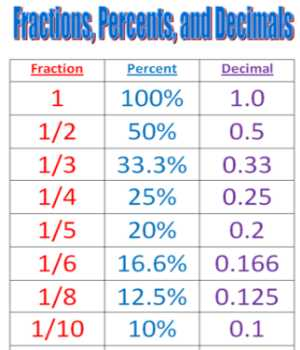Relating Fractions to Equivalent Decimals

To change a fraction to a decimal, you divide the top number by the bottom number (divide the numerator by the denominator). To change a fraction to a decimal, you divide the top number by the bottom number (divide the numerator by the denominator). Some decimals will terminate (end) like the example above, but many will not.

Decimals are a type of fractional number. The decimal 0.5 represents the fraction 5/10. The decimal 0.25 represents the fraction 25/100. Decimal fractions always have a denominator based on a power of 10.

We know that 5/10 is equivalent to 1/2 since 1/2 times 5/5 is 5/10. Therefore, the decimal 0.5 is equivalent to 1/2 or 2/4, etc.

To convert a Fraction to a Decimal manually, follow these steps:

Step 1: Find a number you can multiply by the bottom of the fraction to make it 10, or 100, or 1000, or any 1 followed by 0s.

Step 2: Multiply both top and bottom by that number.

Step 3. Then write down just the top number, putting the decimal point in the correct spot (one space from the right-hand side for every zero in the bottom number).Some common Equivalent Decimals and Fractions:

• 1 and 1/10
• 2 and 1/5
• 5 and ½
• 25 and ¼
• 50 and ½
• 75 and ¾
• 0 and 1/1 or 2/2 or 1

In a fraction, the fraction bar means “divided by.” So to find the decimal equivalent of a fraction like 1/4 you need to solve the math problem: 1 divided by 4.

1 ÷ 4 = 0.25

How to Turn a Fraction into a Division Problem

Dividing numbers is easy with a calculator. If you need to do long division by hand put the top number of the fraction (numerator) inside the division bracket and the bottom number (denominator) outside, to the left of the division bracket.

The fraction 1/4 becomes 1 ÷ 4. Complete the division to convert the fraction to a decimal.

You can reduce the fraction to lowest terms first to make the long division math a bit easier. For example, 9/12 = 9 ÷ 12 = 0.75. Using long division to solve this problem by hand or in your head, reducing 9/12 = 3/4, might make the problem easier. You may even recognize that 3/4 = 0.75 because 3 quarters equals 75 cents.

Information Source;